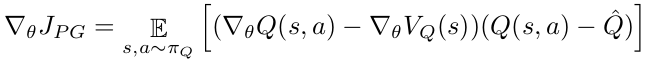# Multi Term Loss for Policy Gradient Algorithm

I’ve been attempting to implement a somewhat exotic Actor-Critic policy gradient algorithm (here’s the paper if you’re interested RL from Imperfect Deomonstrations). The algorithm is similar to DQN, in that we have a network predicting Q values and use a target network to stabilize the results, but instead of updating with mse loss we calculate V from Q and do an update based on the following update equation:This part is tripping me up. How would I go about writing the loss function for this? I can calculate values for the V and Q terms, but I’m not sure how to actually handle something in this form.